﻿ Mechanics Map - Undamped Harmonic Forced Vibrations

# Undamped Harmonic Forced Vibrations

Often, mechanical systems are not undergoing free vibration, but are subject to some applied force that causes the system to vibrate. In this section, we will consider only harmonic (that is, sine and cosine) forces, but any changing force can produce vibration.

When we consider the free-body diagram of the system, we now have an additional force to add, namely the external harmonic excitation.A mass-spring system with an external force, F, applying a harmonic excitation.

The equation of motion of the system above will be:

 $m {\ddot{x}} + k {x} = F$

Where F is a force of the form:

 $F = F_0 \sin \omega_0 t$

This equation of motion for the system can be re-written in standard form:

 ${\ddot{x}} + \frac{k}{m} {x} = \frac{F_0}{m} \sin \omega_0 t$

The solution to this system consists of the superposition of two solutions: a particular solution, xp (related to the forcing function), and a complementary solution, xc (which is the solution to the system without forcing).

As we saw previously, the complementary solution is the solution to the undamped free system:

We can obtain the particular solution by assuming a solution of the form:

 $x_p = D \sin (\omega_0 t)$

Where ω0 is the frequency of the harmonic forcing function. We differentiate this form of the solution, and then sub into the above equation of motion:

 $\ddot{x_p} = - \omega_0^2 D \sin (\omega_0 t)$ $-m \omega_0^2 D \sin (\omega_0 t) + k D \sin (\omega_0 t) = F_0 \sin ( \omega_0 t)$

Solving for D, we find D and the the particular solution, xp:

 $D = \frac\frac{F_0}{k}}{1- \left( \displaystyle \frac{\omega_0}{\omega_n} \right)^2}$ $x_p = \frac\frac{F_0}{k}}{1- \left( \displaystyle \frac{\omega_0}{\omega_n} \right)^2} \sin ( \omega_0 t)$

Thus, the general solution for a forced, undamped system is:

 $x_G(t) = \frac{ \displaystyle \frac{F_0}{k}}{1- \left( \displaystyle \frac{\omega_0}{\omega_n} \right)^2} \sin ( \omega_0 t) + C \sin (\omega_n t + \phi)$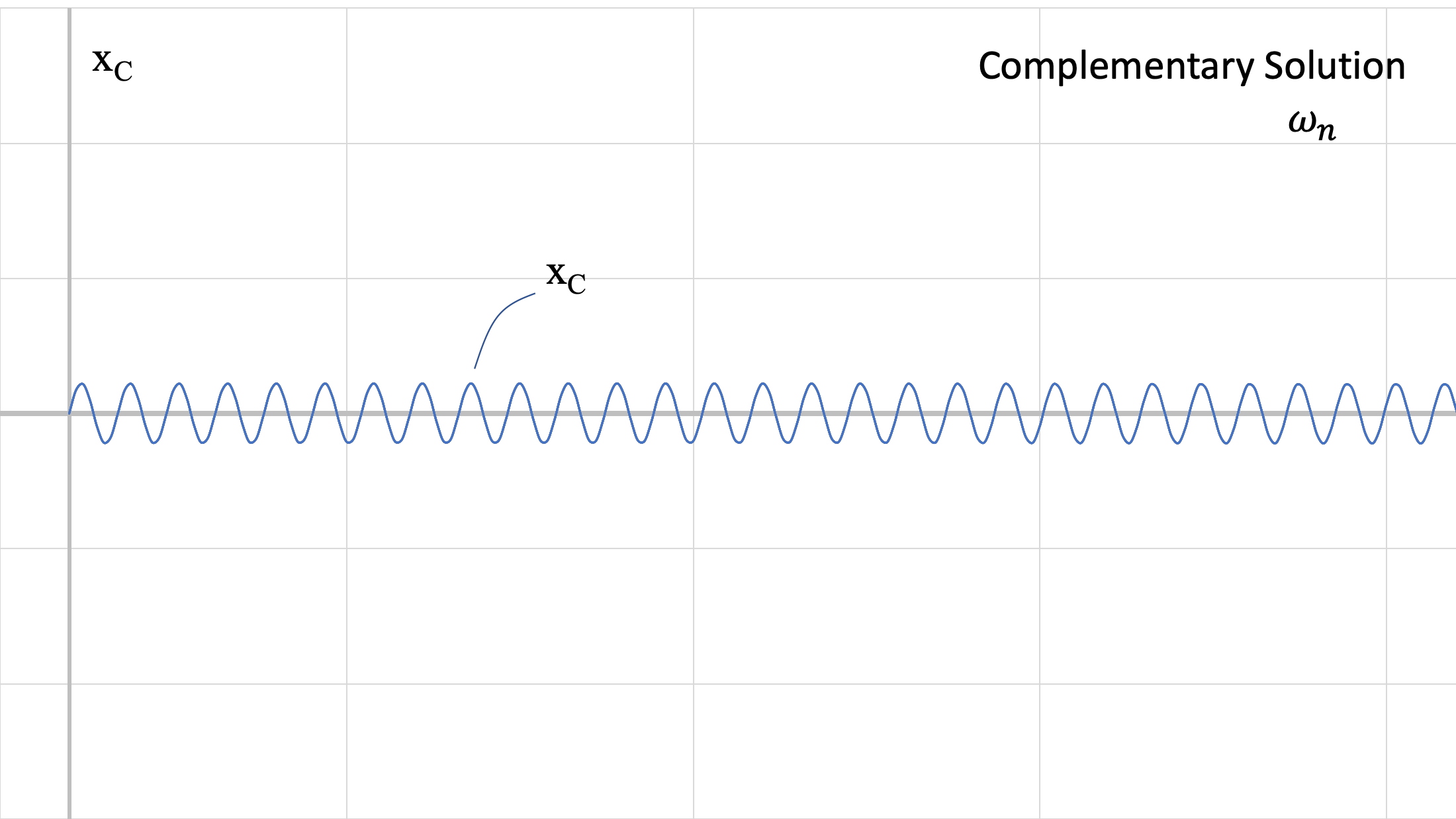The complementary solution of the equation of motion. This represents the natural response of the system, and oscillates at the angular natural frequency. This is the transient response.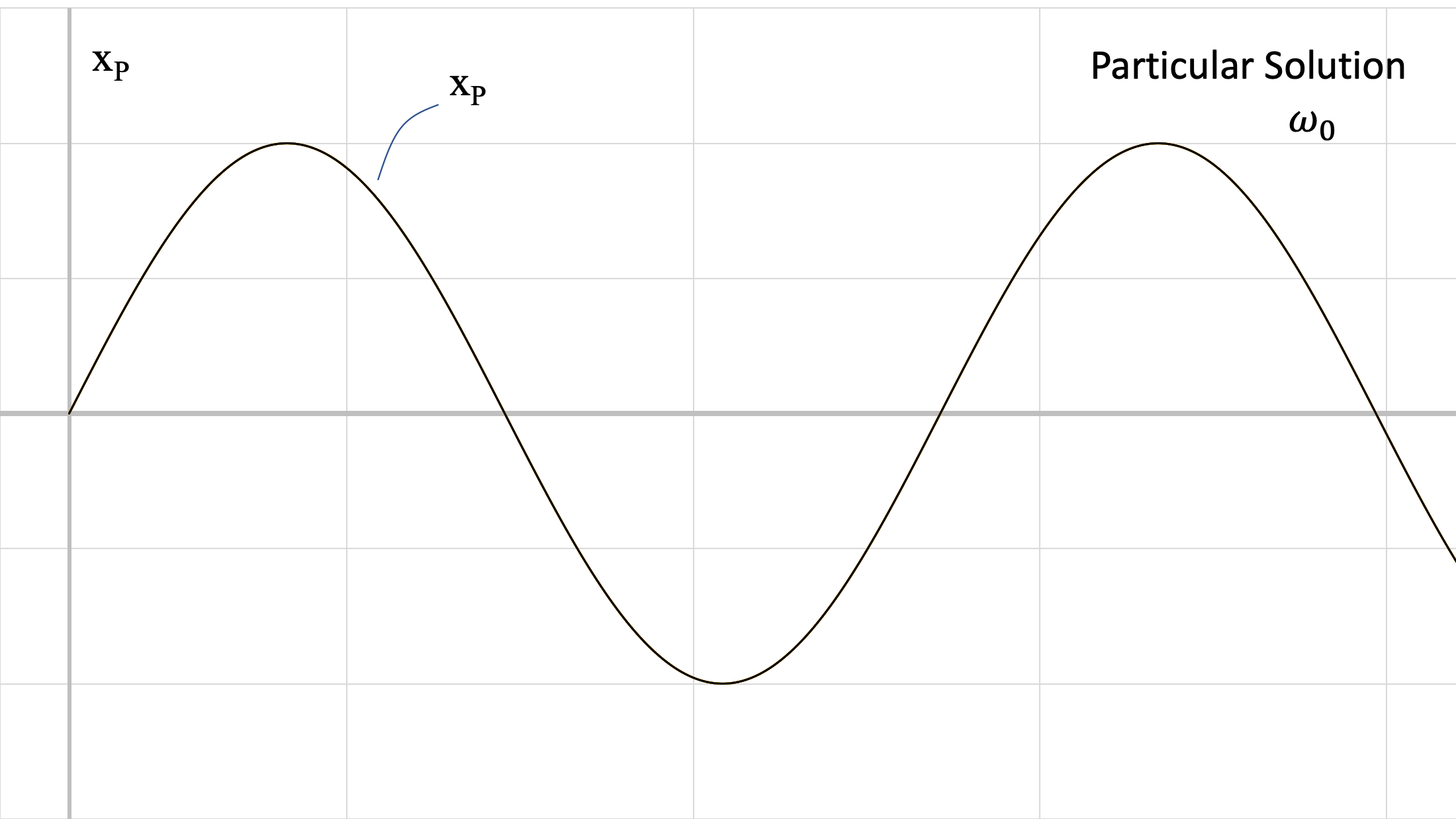The particular solution of the equation of motion. This represents the forced response of the system, and oscillates at the angular forced frequency. This is the steady-state response.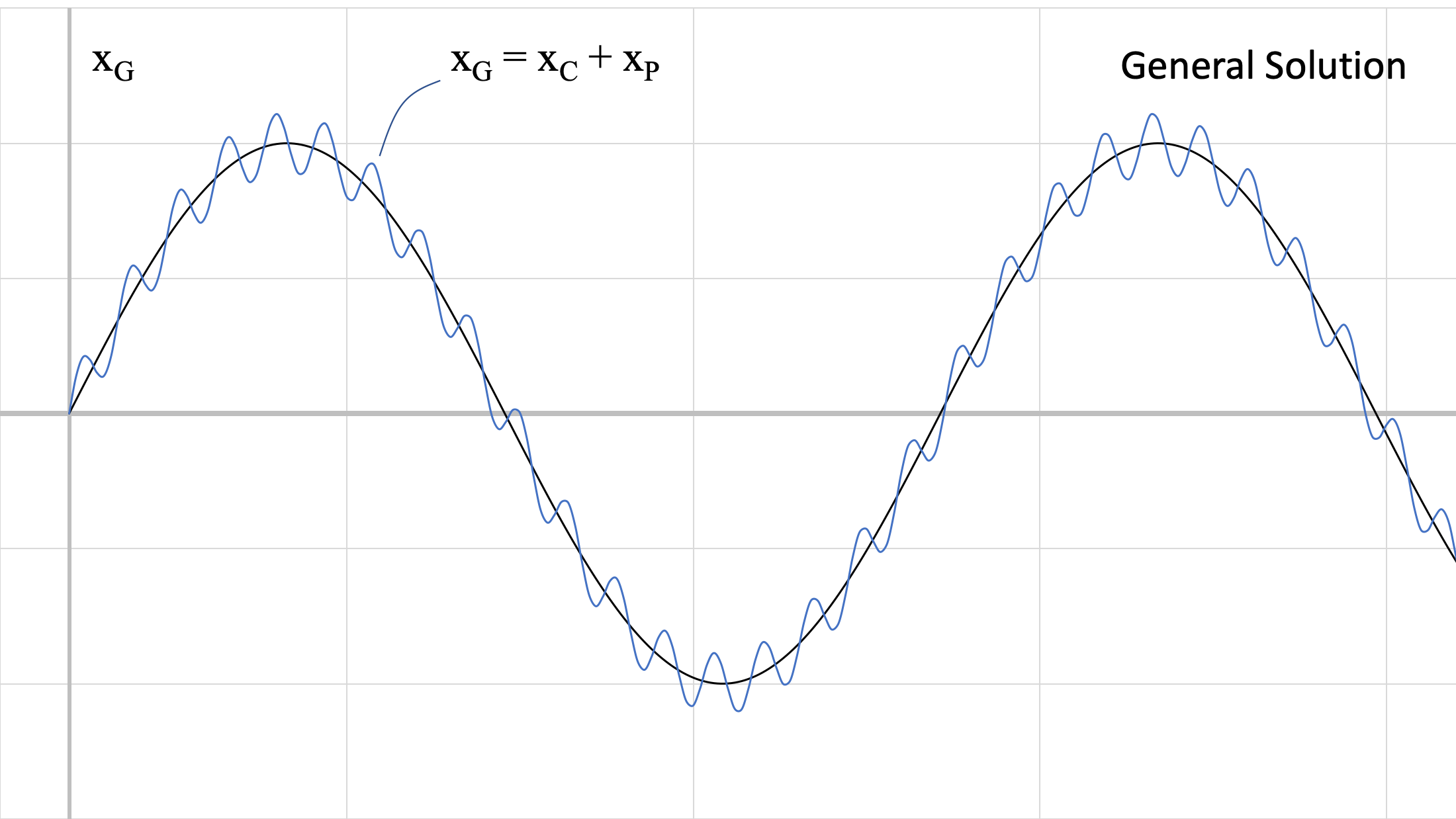The general solution of the equation of motion. This represents the combined response of the system, and the sum of the complementary (or natural) and particular (or forced) responses.

The above figures show the two responses at different frequencies. Recall that the value of ωn comes from the physical characteristics of the system (m, k) and ω0 comes from the force being applied to the system. These responses are summed, to achieve the blue response (general solution) in the third figure.

In reality, this superimposed response does not last long. Every real system has some damping, and the natural response of the system will be damped out. As long as the external harmonic force is applied, however, the response to it will remain. When evaluating the response of the system to a harmonic forcing function, we will typically consider the steady-state response, when the natural response has been damped out and the response to the forcing function remains.

## Amplitude of Forced Vibration

The amplitude of the steady-state forced vibration depends on the ratio of the forced frequency to the natural frequency. As ω0 approaches ωn (ratio approaches 1), the magnitude, D, becomes very large. We can define a magnification factor:

 $MF = \displaystyle \frac{ \frac\frac{F_0}{k}}{1- \left( \displaystyle \frac{\omega_0}{\omega_n} \right)^2}}\frac{F_0}{k}} = \frac{1}{1- \left( \displaystyle \frac{\omega_0}{\omega_n} \right)^2}$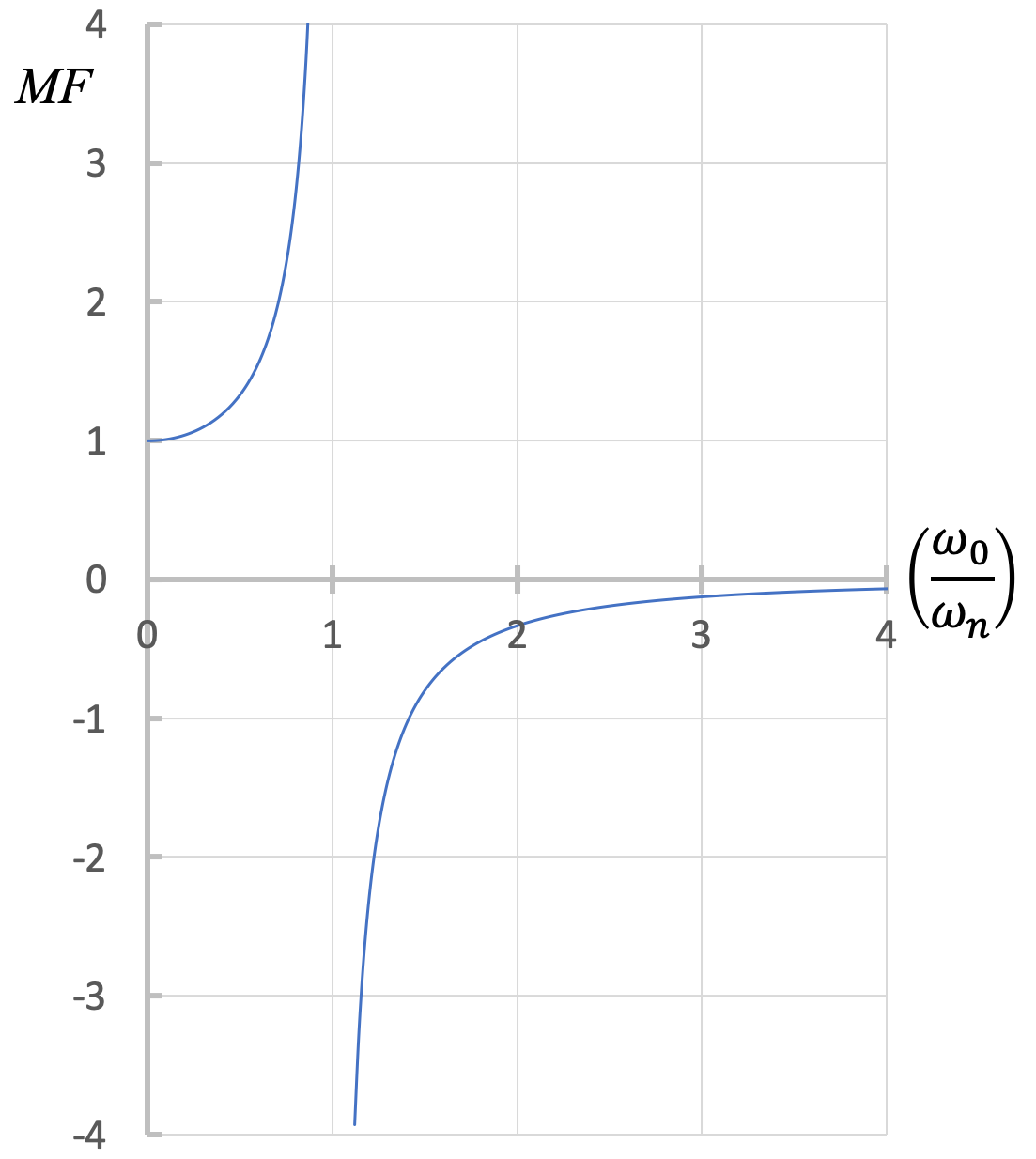The magnification factor, MF, is defined as the ratio of the amplitude of the steady-state vibration to the displacement that would be achieved by static deflection.

From the figure above, we can discuss various cases:

• ω0 = ωn: resonance occurs. This results in very large amplitude vibrations, and is associated with high stress and failure to the system.
• ω0 ~ 0, MF ~ 1: The forcing function is nearly static, leaving essentially the static deflection and limited natural vibration.
• ω0 < ωn: Magnification is positive and greater than 1, meaning the vibrations are in phase (when the force acts to the left, the system displaces to the left) and the amplitude of vibration is larger than the static deflection.
• ω0 > ωn: Magnification is negative and the absolute value is typically smaller than 1, meaning the vibration is out of phase with the motion of the forcing function (when the force acts to the left, the system displaces to the right) and the amplitude of vibration is smaller than the static deflection.
• ω0 >> ωn: The force is changing direction too fast for the block's motion to respond.

## Rotating Unbalance

One common cause of harmonic forced vibration in mechanical systems is rotating unbalance. This occurs when the axis of rotation does not pass through the centre of mass. In this situation, instead of the centre of mass remaining stationary, it experiences some acceleration. This causes a force on the axle that changes direction as the center of mass rotates. We can represent this as a small mass, m, rotating about the axis of rotation at some distance, called an eccentricity, e. The forced angular frequency, ω0, in this case is the angular frequency of the rotating system.

## Worked Problems:

### Question 1:

A 10 kg fan is fixed to a lightweight beam. The static weight of the fan deflects the beam by 20 mm. If the blade is designed to spin at ω = 15 rad/s, and the blade is mounted off-center (equivalent to a 1.5 kg mass at 50 mm from the axis of rotation), determine the steady-state amplitude of vibration.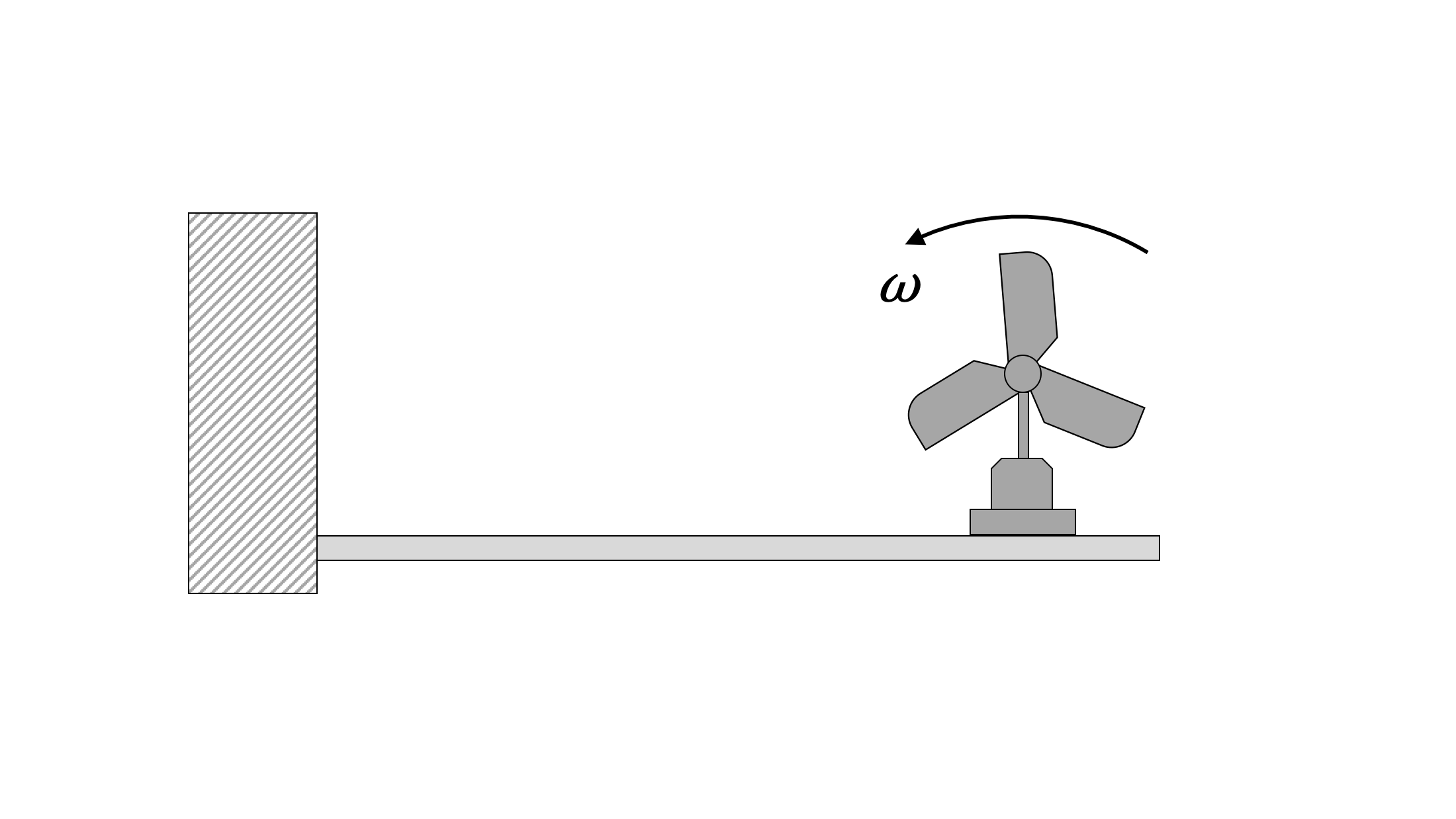### Question 2:

You are designing a stylish fan that uses only one blade. Approximate that blade as a narrow plate with density per unit length of 20g/cm. The base weight of the rest of the device (except for the blade) is 4 kg, and the whole thing is mounted on a lightweight beam. If the spring constant of the beam is k = 1000 N/m, find the length of blade than will cause resonance if the fan is designed to spin at ω = 15 rad/s.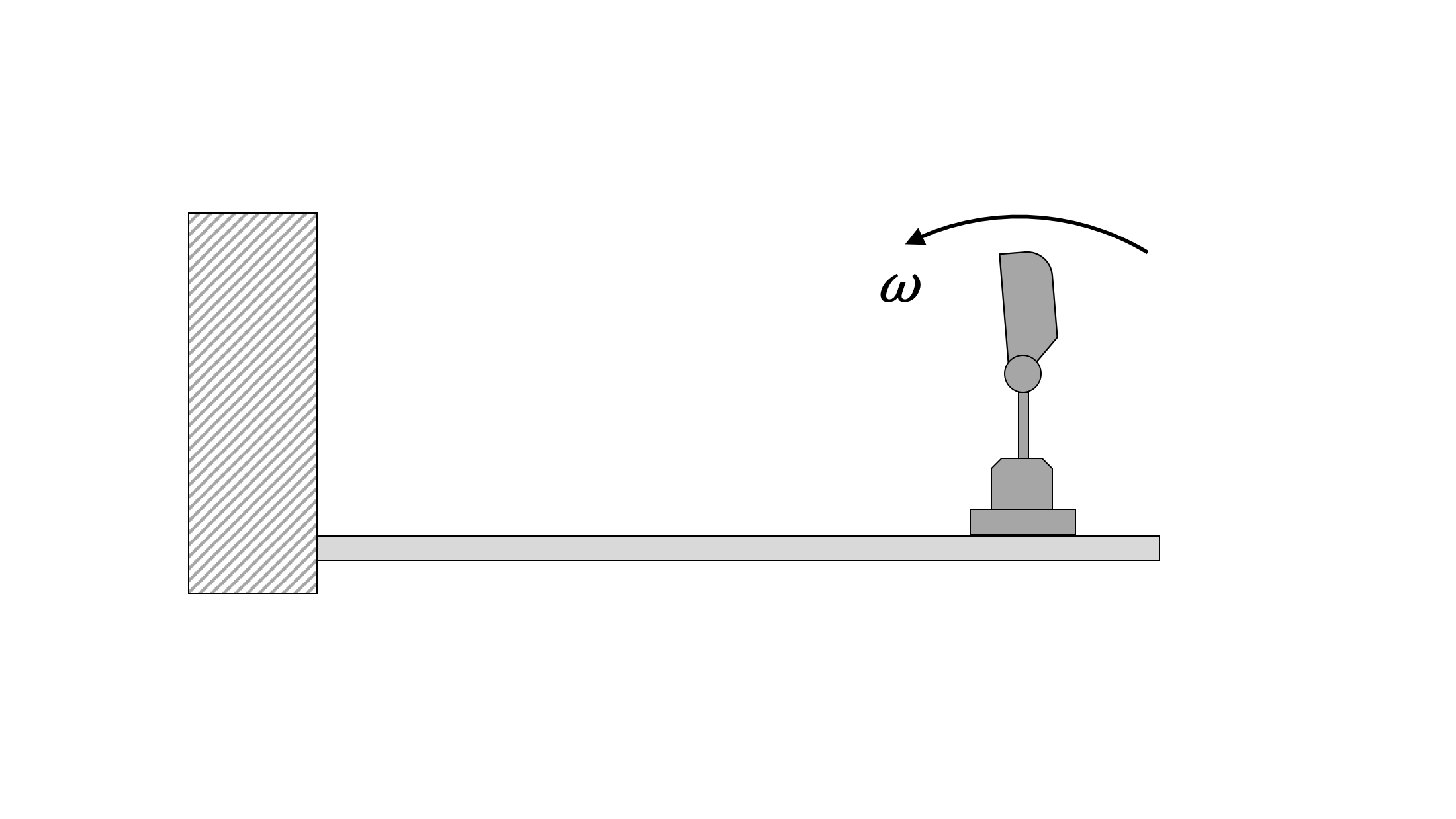### Question 3:

A 2kg box of orange juice contains the instructions “shake well”. An engineering student decides to get smart and connect the juice box with a spring, which has a spring constant k=25N/m, and applies a periodic force. The periodic force is described as F=5sin⁡(5t). What is the maximum amplitude of the periodic motion and Magnification Factor at steady state?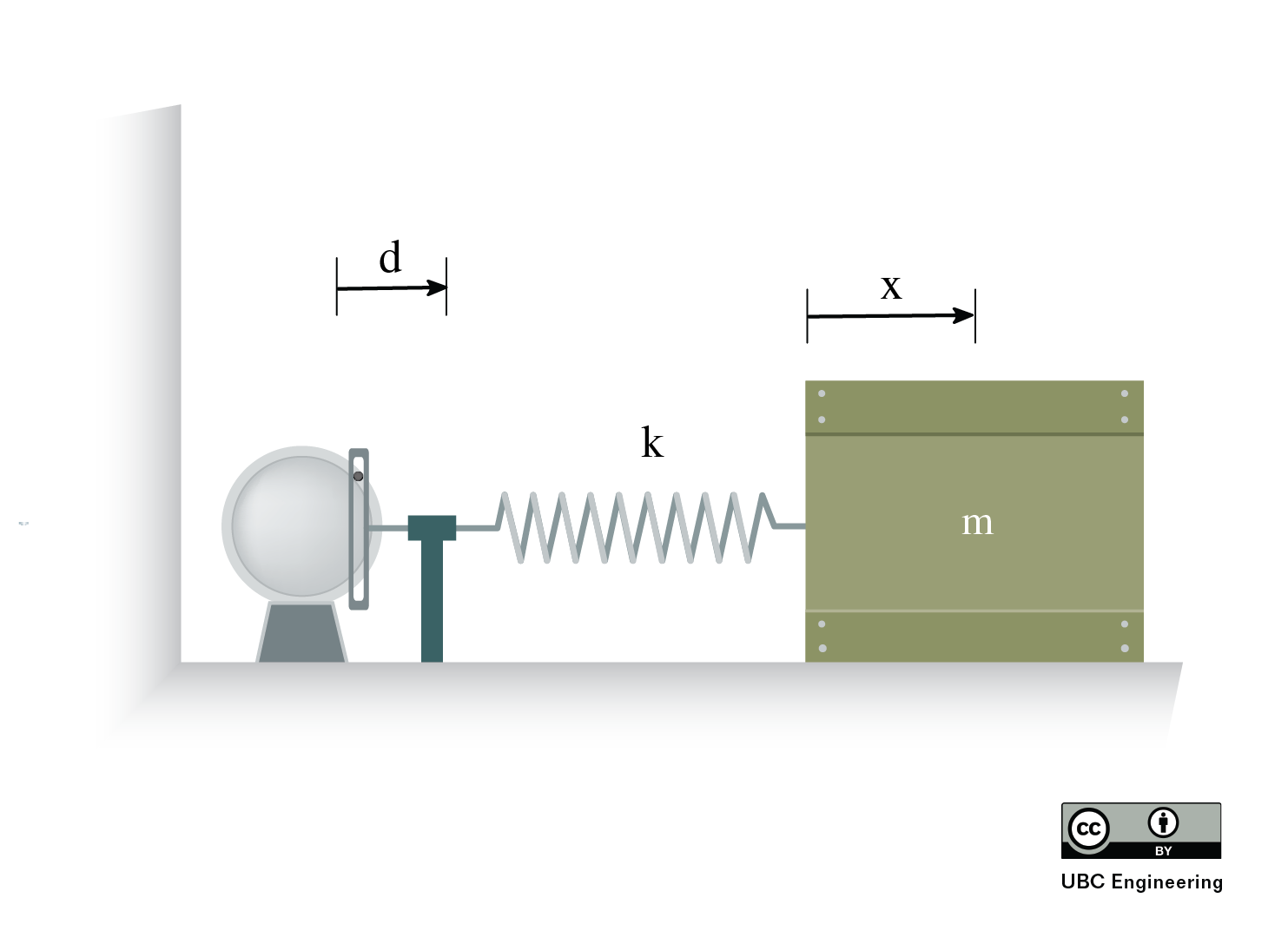### Question 4:

The world’s smallest Ferris wheel (m=20kg) is mounted on the end of a horizontal beam for no apparent reason. The wheel is mounted eccentrically in such a way that the eccentricity is equivalent to a mass of 5kg located 0.15m away from the axis of rotation. The static weight of the Ferris wheel causes a deflection of 20mm in the beam. Given that the wheel spins at a rate of 15 rad/sec, find the steady-state amplitude of vibration.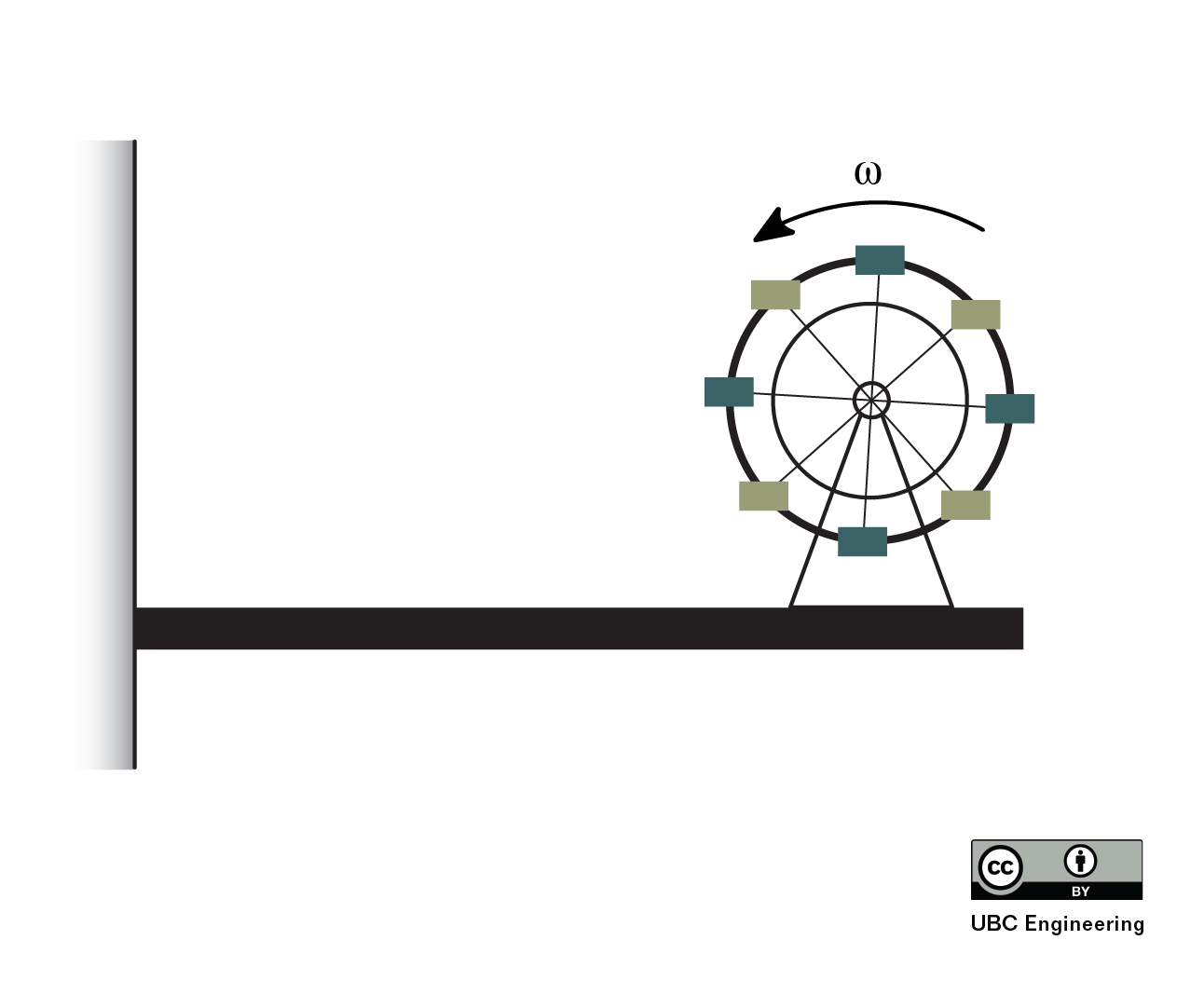### Question 5:

A 150 kg mass is supported on three springs. Find the spring constant, k [N/m], required for a magnification factor of 2 when F(t) = 25 sin(4t).